## How to draw a streamline by using stream function ...

How to draw a streamlines by using stream function provided in pdf. In the presence and absence of lambda parameter.

## Polynomials: How do I determine the linear factors...

Show that x-2 is a factor of p(x)=x^3+3x^2-4x-12

I)determine all the linear factors of p(x)

ii)what are the zeros of y=p(x)

iii)sketch function y=p(x)

## Is there a more versatile way to draw the curve on...

I read the code in the link below and have some thoughts on drawing the graph.

https://www.mapleprimes.com/questions/216092-Options-For-Graph-Drawing-In-The-GraphTheory

restart:
with(GraphTheory):
with(SpecialGraphs):
G     := PetersenGraph();:
POS := GetVertexPositions(G);
EG   := Edges(G);
PLOT(
seq( CURVES([POS[EG[i]], POS[EG[i]]], LINESTYLE(3) ), i=1..numelems(Edges(G))),
POINTS(POS, SYMBOL(_SOLIDBOX, 35), COLOR(RGB, 1, 1, 0)),
seq(TEXT(POS[i], i), i=1..numelems(Vertices(G))),
AXESSTYLE(NONE)
)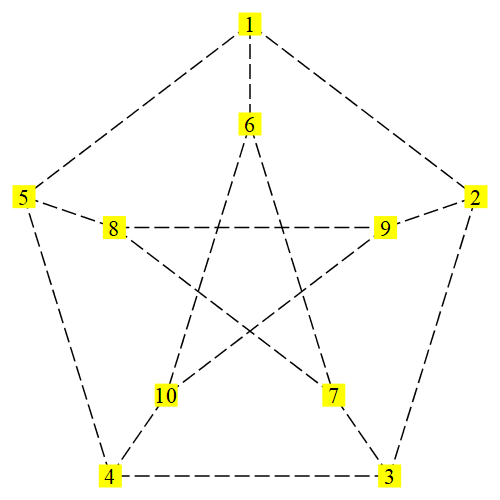But if we want to change the edge to a curvilinear style, it seems very difficult. We know  PetersenGraph is 1-planar graph.  The following picture is one of  its 1-plane drawing.  That is  also what I want to draw .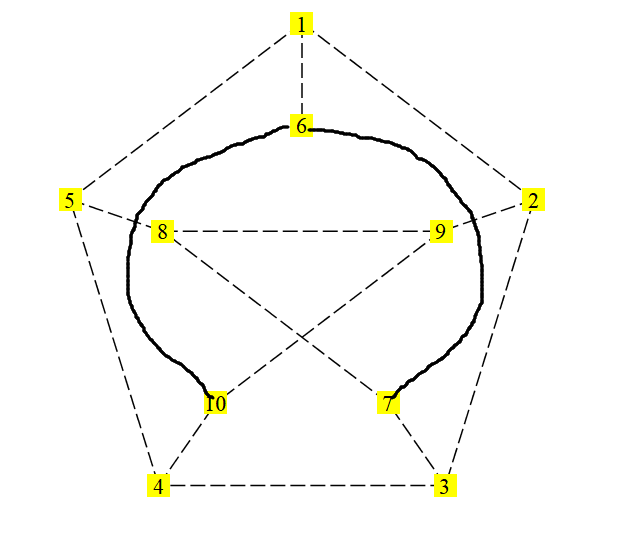PS: 1-planar graph is a graph that can be drawn in the Euclidean plane in such a way that each edge has at most one crossing point, where it crosses a single additional edge

Although I asked a similar question, but it uses too many special curve functions and the method is relatively isolated. Because the cycle graph is too special.  https://www.mapleprimes.com/questions/228987--Can-We-Draw-Curved-Edges-In-The-Graph

I also noticed the optional items of the edge style.  But there are no curves, such as arcs, parabola, etc.

The plot style must be one of line, point, pointline, polygon (patchnogrid), or polygonoutline (patch).

I am trying to draw a parabola, it seems to be quite difficult. And it completely deviated from the use of graph theory package.

drawarc :=proc(A,B,C,ecolor);

local  c,ax,cx ,ay,cy,ox,oy,oo,x,y,b,a,an_end,an_start,r,yuanhu:
ax :=evalf(geometry)[HorizontalCoord](A):
cx :=evalf(geometry)[HorizontalCoord](C):
ay :=evalf(geometry)[VerticalCoord](A):
cy :=evalf(geometry)[VerticalCoord](C):

geometry[circle](c,[ A ,B ,C], [x,y],'centername' =o):

ox :=evalf(geometry[HorizontalCoord] (o)):
oy :=evalf(geometry[ VerticalCoord] (o)):

if(cx -ox)>0 then
b :=arctan((cy -oy) /(cx -ox)):
elif(cx -ox)=0 and (cy -oy)>0 then

b :=Pi/2 :
elif(cx -ox)=0 and (cy -oy)<0 then

b :=-Pi/ 2 :
else
b :=Pi +arctan((cy -oy) /(cx -ox)):
fi :
if(ax -ox)>0 then
a :=arctan((ay -oy) /(ax -ox)):
elif(ax -ox)=0 and (ay -oy)>0 then

a :=Pi /2 :
elif(ax -ox)=0 and (ay -oy)<0 then

a :=-Pi/ 2 ;
else
a :=Pi +arctan((ay -oy)/ (ax -ox)):
fi ;
if(evalf(b)<evalf(a))then
an_start :=a :
else
an_start :=a +2*Pi :
fi :
an_end :=b :

yuanhu :=plottools[ arc] ([ ox , oy] , r , an_start..an_end):

plots[ display] (yuanhu , scaling =constrained, color =ecolor,axes=none);

end :

geometry[point] (a , -3 .00 , 1 .70);
geometry[point] (b , 0 .35 , -0 .45);
geometry[point] (c , 3 .13 , 1 .86);
l :=[a , b , c] ;
drawarc(a,b,c,blue);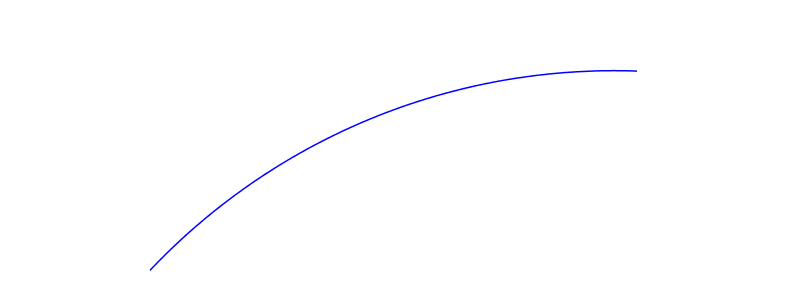I would like to ask maple if there is a more versatile and simpler way to draw a curve of  graph drawing.

drawarcs:=proc(VL ::list , ecolor , scope);
local i , num , arcs , arc_text , vl , display_set ;
vl :=VL ;
num:=nops(vl);
arcs :={};
i:=1;
if num <3 then
"There isn' t enough points in the point list." ;
return ;
elif irem(num-1 , 2)<>0 then
"The number of the list must be multiple of 2 when it minus 1 .";
fi :
while i <num do
arcs :={drawarc(vl[i] ,vl[i +1] , vl[i +2],ecolor),op(arcs)};
i:=i +2 ;
od ;
arc_text :=geometry[ draw] ({vl , vl[num] }, printtext =true , color =ecolor);
display_set:={op(arcs),arc_text};
plots[ display] (display_set , view =[ -abs(scope)..abs(scope), -abs(scope)..abs(scope)] , scaling =constrained,axes=none);
end proc:
geometry[ point] (v1 , 0 ,0):
geometry[ point] (a , 3, 9):
geometry[ point] (b , 7, 9):
geometry[ point] (c, 6,8):
geometry[ point] (d , 12,9):
geometry[ point] (e , 7 ,2):
geometry[ point] (v3 , 9 ,0):
vl:=[ v1,a,b,c,d,e,v3] :
drawarcs(vl,red,20);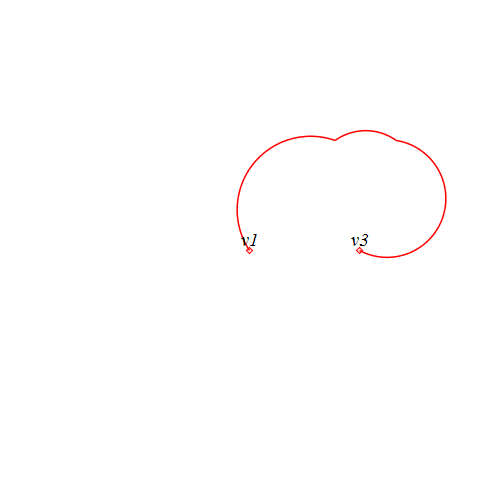try.mwtry.mw

The above program is too cumbersome and not robust

macro(GS=GetVertexPositions):
with(GraphTheory):
with(SpecialGraphs):
G     := PetersenGraph():
POS := GetVertexPositions(G):
EG   := Edges(G) minus {{6,10},{6,7}}:
s1:=PLOT(
seq( CURVES([POS[EG[i]], POS[EG[i]]], LINESTYLE(3) ), i=1..numelems(Edges(G))-2),
POINTS(POS, SYMBOL(_SOLIDBOX, 35), COLOR(RGB, 1, 1, 0)),
seq(TEXT(POS[i], i), i=1..numelems(Vertices(G))),
AXESSTYLE(NONE)
):
geometry[ point] (a,op(GS(G))):
geometry[ point] (b , op(GS(G))):
geometry[ point] (c , op(GS(G))):
geometry[ point] (d ,op(GS(G))+0.3,op(GS(G))-0.5):
geometry[ point] (d2 ,op(GS(G))-0.3,op(GS(G))-0.5):
s2:=drawarc(a,d,b,red):
s3:=drawarc(c,d2,a,red):
plots:-display({s1,s2,s3});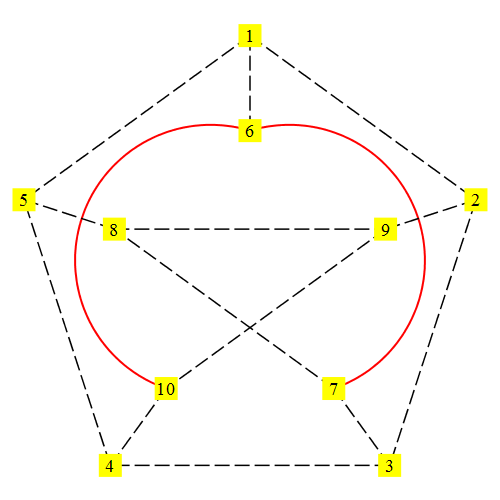## How do I search for maxima on a polygon constraint...

So in multivariate calculus there is a function called Lagrange Multipliers that searches for maxima, minima and saddle points on a 3d surface with an algebraic as a constraint. I was wondering if there was a way to do something similar on a polygon constraint by feeding the corner points to a procedure and returning all extrema and saddle points? Ideas I've had so far is using the directional derivative on the corners in the direction towards the next corner and looking if it is bigger or equal than 0 if multiplied with the directional derivative towards the previous corner (if yes, extrema or saddle point, if no not) however this adresses only corners and there may be extrema on the lines between the corners too. What should I do for that?

## How to use modular arithmetic in Maple?...

Hi,

Is there a way to make all computations in Maple to run modulo specified prime p? If, for example, I want to use a function such as DifferentialAlgebra:-RosenfeldGroebner and force it to run its internal computations modulo p, can this be done?

So far I have tried to use GF(p, k), but there seem to be issues where `+` and other operations require defining all symbold via :-ConvertIn().

Thanks.

## why my question have been deleted...

Dear administration, I hope you all are good,why my question have been deleted? My question is a little similar to the one before, but there are still a lot of differences.I didn't do a backup which was bad.Once deleted, there is no chance of modification.

## Grading Package, Is there a possibility of alignin...

Hi,

Is there a possibility of aligning choices in a horizontal manner?

Thanks

QuizzTest1.mw

## something wrong with a function in a procedure...

Hello!

I have a difficulty with a function used in procedure. The procedure uses a multivariable function and if the specific choice of the function is not made the procedure seems to give proper result, but In case I make a specific choice of the function and then try use this procedure gives me incorrect result.

To be more exact I use Physics package (there is a need to calcute combinations of covariant derivates ). The calculations are performed in a curved space with a defined metric.

So here is the procedure:

SD2 := proc (psi) SumOverRepeatedIndices(g_[`~kappa`, `~lambda`]*(d_[kappa](d_[lambda](psi(X)))-Christoffel[`~sigma`, kappa, lambda]*d_[sigma](psi(X))))^2-SumOverRepeatedIndices(g_[`~kappa`, `~rho`]*(d_[kappa](d_[lambda](psi(X)))-Christoffel[`~sigma`, kappa, lambda]*d_[sigma](psi(X)))*g_[`~lambda`, `~tau`]*(d_[rho](d_[tau](psi(X)))-Christoffel[`~gamma`, rho, tau]*d_[gamma](psi(X)))) end proc;

If I turn to the procedure :

SD2(psi);

the result is  correct.

But  then I specify a psi function:

psi:=(t,r,x,y,z)->chi(r)+q*t;(here q is supposed to be a constant)

and turn to the procedure once again:

SD2(psi);

It gives me a wrong result.

I don't know what is the reason.

Thank you.

## Multiple people writing in the same document...

Hello, I'm a student in high-school, and i was wondering if it is possible to have multiple people writing in the same document at the same time. It would make groupwork a lot easier if I could do the writing like e.g.: google docs.
-Max

## SubString with special characters...

Here's another possible bug related to special characters (like ø, æ, å).

SubString considers each of the characters to be consisting of 2 different other ones.

Related to https://www.mapleprimes.com/questions/231765-Special-Characters-In-Maple-Workbook-Code.(1)(2)(3)(4)## Disk partition and memory...

Hi,

I'm using Maple on a linux partition and for some time now I can't really use it anymore. For example, when I open a help window, I can't scroll through the content, and scrolling gets stuck if an image needs to be displayed. I think my partition is too full and does not have enough free space left. Doesn't Maple create a temporary file that is too big for the space available?  But I have another partition that is not in the same format as the linux partition but is nevertheless readable and writable. Is it possible, if the problem is a temporary file, that this file is written to this other partition to avoid this storage problem?
Or could it be due to a memory problem (RAM?) that did not occur before?

## partial differential equations, PDEs...

Hi,

I want to solve this system of PDEs. Please let me know how I can solve it.

Kindly find both my code written in Maple and the image of the problem.

Best,PDE.mw

## Deleted questions again...

Two days ago I have answered a question about using numapprox:-infnorm for functions of several variables. The question is now deleted. Is it possible to find whether it was deleted by the author (even if she/he has used the "best answer" tag)?

## System of PDE's...

PDE:

(a+b)*(uxx+vxy)+c*laplacian(u)=0

(a+b)*(vxy+vyy)+c*laplacian(v)=0

a,b,c=const.  Initial:u=1/2*sin(2*theta),v=cos(theta)

Text book says: Solve by complex variable method

## How to manage regionalized language in Maplets:-El...

Hi,

I'm french and I'm working on an Imac.

The path for the desktop is  /Users/myname/Desktop.
If cur := "/Users/myname", one can easily verify this:

```IsDirectory(cat(cur, "/Desktop"))
true
```

In some application I'm developping, I use a  FileDialog Maplet to select a directory where to write a file.
For an unknown reason this maplet doesn't display the desktop with the name Desktop, but with it's french counterpart Bureau.

```# Observe thet the desktop (8th line above) is named "Desktop"
# Now run

maplet := Maplet(
FileDialog['FD1'](
'title' = "Output Directory",
'directory' = cur,
'fileselectionmode' = directoriesonly,
'onapprove' = Shutdown(['FD1']),
'oncancel' = Shutdown()
)
):

res := Maplets[Display](maplet):
StringTools:-StringSplit(res[], cur);

["", "/Bureau"]
```As a result, saving a file in cur/Bureau generates an error.
Here is a more detailed worksheet:
English_French_confusion.mw

Of course I have adjusted my code in an ad hoc way by french translating  what the maplet returns, but it is just a band-aid on a larger wound. For instance "Downloads" appears as "Téléchargements", and so on, and many names potentially need to be translated.

I do not understand why FileTools seems to "work in english" while  FileDialog seems to "work in french".
I suspect this is due to the fact that my Imac, although natively "English speaking", uses a French overlay.
For instance the  Unix command pwd run in a terminal window indicates Users or Desktop, while  < command + i > executed on a given file displays Utilisateurs and Bureau.
Could it be that FileTools relies upon English, while FileDialog  relies upon the regionalized language?